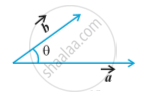Product of Two Vectors - Scalar (Or Dot) Product of Two Vectors

definition

The scalar product of two nonzero vectors vec a and vec b, denoted by vec a .vec b , is defined as vec a . vec b = |vec a| |vec b| cos theta,
where ,  θ is the angle between Fig.If either vec a = 0 or vec b = 0 then θ is not defined, and in this case , we define vec a . vec b = 0

Observations:
1) vec a .vec b is a real number.

2) Let vec a and vec b be two nonzero vectors, then vec a . vec b = 0 if and only if vec a and vec b are perpendicular to each other . i.e.
vec a . vec b = 0  <=> vec a ⊥ vec b

3)  If θ = 0, then vec a .vec b = |vec a| |vec b|
In particular , vec a .vec a = |vec a|^2 , as θ in this case is 0.

4) If θ = π, then vec a . vec b = - |vec a||vec b|
In particular, vec a . vec b = - |vec a||vec b|, as θ in this case is π.

5)  In view of the Observations 2 and 3, for mutually perpendicular unit vectors
hat i , hat j   "and"   hat k we have
hat i . hat i = hat j . hat j = hat k. hat k = 1,
hat i . hat j = hat j . hat k = hat k. hat i = 0

6) The angle between two nonzero vectors vec a and vec b is given by
cos theta = (vec a .vec b)/(|vec a||vec b|), or theta = cos ^(-1)  ((vec a . vec b)/(|vec a||vec b|))

7) The scalar product is commutative. i.e.
vec a . vec b = vec b .  vec a

text

Two important properties of scalar product :

Property:
(Distributivity of scalar product over addition)
Let vec a,  vec b and vec c be any three vectors , then vec a (vec b + vec c) = vec a . vec b + vec a. vec c

Property:
Let vec a and vec b be any two vectors, and l be any scalar. Then
(lambda vec a). vec b = (lambda vec a).vec b = lambda (vec a . vec b) = vec a . (lambda vec b)
If two vectors vec a  and  vec b are given in component form as

a_1 hat i + a_2hat j + a_3 hat k  and  b_1 hat i + b_2hat j + b_3 hat k .,  then their scalar product is given as
vec a . vec b = (a_1hat i + a_2 hat j + a_3 hat k) . (b_1 hat i + b_2 hat j + b_3 hat k)
= a_1hat i . (b_1 hat i + b_2 hat j + b_3 hat k) + a_2 hat j . (b_1 hat i + b_2 hat j + b_3 hat k) + a_3 hat k . (b_1 hat i + b_2 hat j + b_3 hat k)
= a_1 b_1 (hat i . hat i) + a_1b_2 (hat i . hat j) +a_1b_3 (hat i . hat k)+a_2b_1 (hat j . hat i) + a_2 b_2 (hat j . hat j) + a_2b_3 (hat j . hat k) + a_3b_1 (hat k . hat i) + a_3b_2 (hat k . hat j) + a_3b_3 (hat k . hat k)   (Using the above Properties 1 and 2)
= a_1b_1 + a_2b_2 + a_3b_3                             (Using Observation 5)

Thus vec a . vec b = a_1b_1 + a_2b_2 + a_3b_3

If you would like to contribute notes or other learning material, please submit them using the button below.

Video Tutorials

We have provided more than 1 series of video tutorials for some topics to help you get a better understanding of the topic.

Series 1

Series 2

Shaalaa.com

DOT PRODUCT [00:38:10]
S
0%